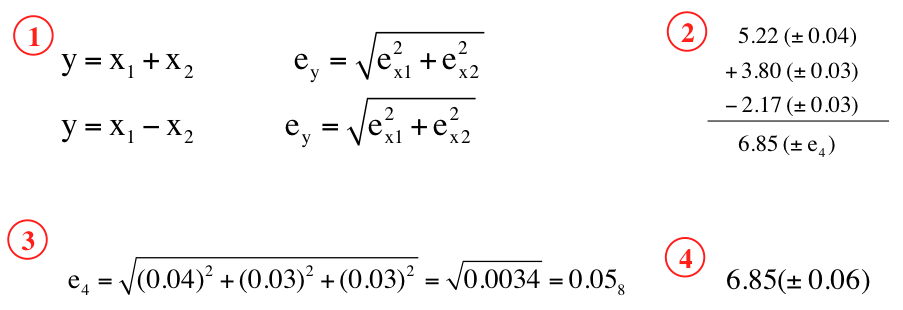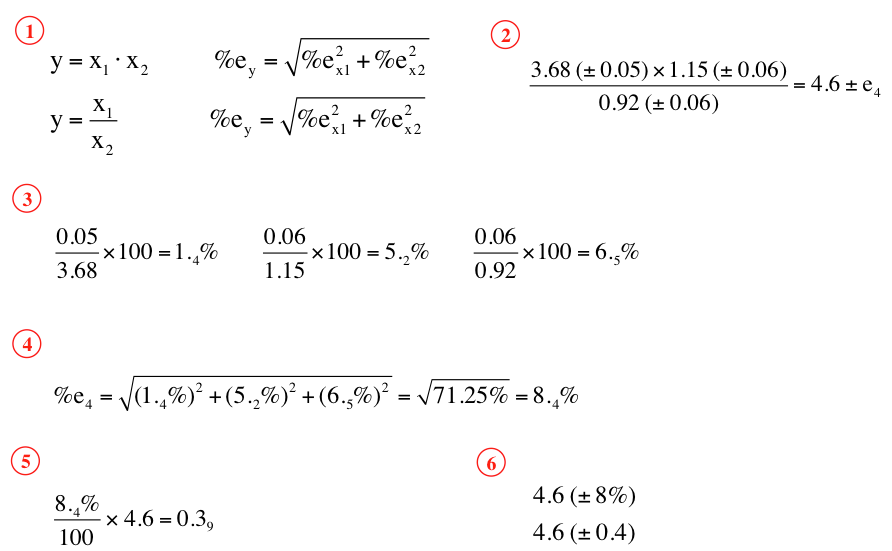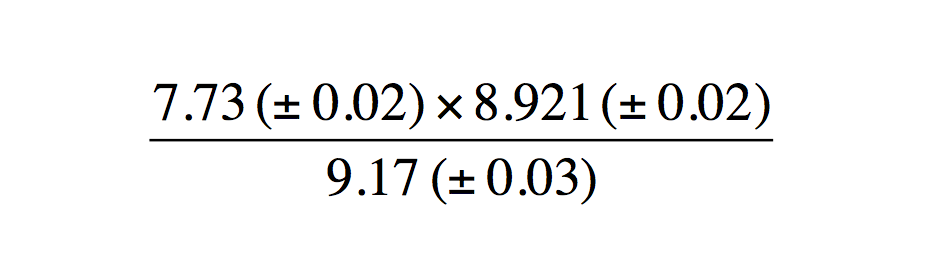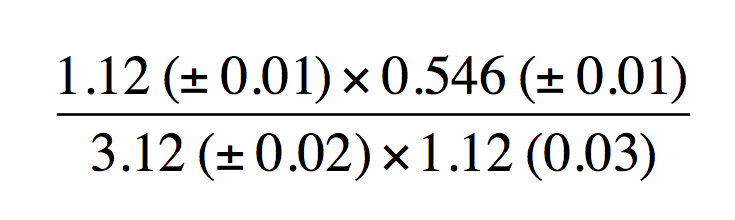Clutch Prep is now a part of Pearson
Ch.3 - Experimental ErrorWorksheetSee all chapters

# Uncertainty

See all sections
Sections
Significant Figures
Addition and Subtraction Operations
Multiplication and Division Operations
Logarithm and Anti-Logarithm Operations
Precision and Accuracy
Types of Errors
Uncertainty
Propagation of Uncertainty: Triangular Distribution

Uncertainty can be thought of as the range (+/-) that is associated with any given value.

###### Types of Uncertainty

Concept #1: With any given value there is some level of uncertainty, which can be classified as absoluterelative or percent​.

###### Expert Q&A

Ask unlimited questions and get expert help right away.

Example #1: Calculate the relative and percent relative uncertainty from the given problem.

3.25 (± 0.03)

###### Expert Q&A

Ask unlimited questions and get expert help right away.

Practice: Calculate the absolute uncertainty from the given problem.

6.77 (± 5.6%)

###### Propagation of Uncertainty from Random Error

Concept #2: With any calculations dealing with uncertainty we will have to take into account the Real Rule

###### Expert Q&A

Ask unlimited questions and get expert help right away.

Concept #3: For addition and subtraction, the uncertainty in our final answer is determined from each individual absolute uncertainty.

###### Expert Q&A

Ask unlimited questions and get expert help right away.Example #2: For multiplication and division, we must first convert the absolute uncertainties into percent relative uncertainties.

###### Expert Q&A

Ask unlimited questions and get expert help right away.Example #3: Determine the absolute and relative uncertainty to the following addition problem.

1.511 (± 0.02) + 2.53 (± 0.01) + 0.987 (± 0.01)

###### Expert Q&A

Ask unlimited questions and get expert help right away.

Practice: Determine the absolute and relative uncertainty to the following addition and subtraction problem.

8.88 (± 0.03) - 3.29 (± 0.10) + 6.43 (± 0.001)

Example #4: Determine the absolute and relative uncertainty to the following multiplication and division problem.

###### Expert Q&A

Ask unlimited questions and get expert help right away.Practice: Determine the absolute and relative uncertainty to the following multiplication and division problem.

1.12(±0.01) x 0.546 (±0.01) / 3.12(±0.02) x 1.12 (0.03)Example #5: Two students wish to prepare a stock solution for their lab experiment. Student A uses an un-calibrated pipet that delivers 50.00 (± 0.02) mL to deliver 200 mL to a container. Student B uses a calibrated pipet that delivers 40.00 (± 0.01) mL to deliver 200 mL to a container.

a) Calculate the absolute uncertainty in each of their deliveries.

###### Expert Q&A

Ask unlimited questions and get expert help right away.

Practice: Based on the previous example calculate the molarity value for each student if they dissolve 0.300 (± 0.03) moles of analyte.

Example #6: A “Class A” 50-mL buret is certified by the manufacturer to deliver volumes within a “tolerance” (i.e. uncertainty) of ± 0.05 mL.  The smallest graduations on the buret are 0.1 mL.  You use the buret to titrate a solution, adding 5 successive volumes to the solution.  The following volumes were added:

1                  6.73

2                  8.92

3                  7.52

4                  2.48

5                  5.15

What is the total volume added, and what is the uncertainty associated with this final volume?

###### Expert Q&A

Ask unlimited questions and get expert help right away.

Example #7: A Class A 250 mL volumetric flask has an uncertainty of ± 0.15 mL, and a 50 mL volumetric pipet has an uncertainty of ± 0.05 mL. If I fill a 250 mL volumetric flask to the line and remove four 50 mL aliquots with my volumetric pipet, I should have 50 mL of solution remaining in the flask. What is the absolute and relative uncertainty in the remaining volume?

###### Expert Q&A

Ask unlimited questions and get expert help right away.

Practice: I am making a 0.1 M KCl (molar mass 74.551) solution for an experiment. To measure the mass of the KCl, I will use an analytical balance that is only accurate to ± 0.01 g. I place a piece of paper on the balance and set the tare to read 0.00. I then put the KCl on the balance until it reads 6.79 g. What is the uncertainty in this mass?

Practice: The volume of the solution I am making is 2.5 L. To measure this volume I will use a large graduated cylinder that can measure volume to ± 10 mL. What is the absolute uncertainty in my concentration?

###### Expert Q&A

Ask unlimited questions and get expert help right away.